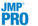Basic Analysis > Oneway Analysis > Nonparametric Tests
Publication date: 07/30/2020

# Nonparametric Tests

Nonparametric tests are useful when the usual analysis of variance assumption of normality is not viable. The Nonparametric options provide several methods for testing the hypothesis of equal means or medians across groups. Nonparametric multiple comparison procedures are also available to control the overall error rate for pairwise comparisons. Nonparametric tests use functions of the response ranks, called rank scores. See Hajek (1969) and SAS Institute Inc. (2017a).

Note the following:

For the Wilcoxon, Median, Van der Waerden, and Friedman Rank tests, if the X factor has more than two levels, a chi-square approximation to the one-way test is performed.

If you specify a Block column, the nonparametric tests (except for the Friedman Rank Test) are conducted on data values that are centered using the block means.

Wilcoxon Test

Performs a test based on Wilcoxon rank scores. The Wilcoxon rank scores are the simple ranks of the data. The Wilcoxon test is the most powerful rank test for errors with logistic distributions. If the factor has more than two levels, the Kruskal-Wallis test is performed. For information about the report, see The Wilcoxon, Median, Van der Waerden, and Friedman Rank Test Reports. For an example, see Example of the Nonparametric Wilcoxon Test.

The Wilcoxon test is also called the Mann-Whitney test.

Median Test

Performs a test based on Median rank scores. The Median rank scores are either 1 or 0, depending on whether a rank is above or below the median rank. The Median test is the most powerful rank test for errors with double-exponential distributions. For information about the report, see The Wilcoxon, Median, Van der Waerden, and Friedman Rank Test Reports.

van der Waerden Test

Performs a test based on Van der Waerden rank scores. The Van der Waerden rank scores are the ranks of the data divided by one plus the number of observations transformed to a normal score by applying the inverse of the normal distribution function. The Van der Waerden test is the most powerful rank test for errors with normal distributions. For information about the report, see The Wilcoxon, Median, Van der Waerden, and Friedman Rank Test Reports.

Kolmogorov Smirnov Test

(Available only when the X factor has two levels.) Performs a test based on the empirical distribution function, which tests whether the distribution of the response is the same across the groups. Both an approximate and an exact test are given. For information about the report, see Kolmogorov-Smirnov Two-Sample Test Report.

Friedman Rank Test

(Available only when a Block variable is specified in the launch window.) Performs a test based on Friedman Rank scores. The Friedman Rank scores are the ranks of the data within each level of the blocking variable. The parametric version of this test is a repeated measures ANOVA. For information about the report, see The Wilcoxon, Median, Van der Waerden, and Friedman Rank Test Reports.

Note: There must be an equal number of observations within each block.Exact Test

Provides options for performing exact versions of the Wilcoxon, Median, van der Waerden, and Kolmogorov-Smirnov tests. These options are available only when the X factor has two levels. Results for both the approximate and the exact test are given.

For information about the report, see 2-Sample, Exact Test. For an example involving the Wilcoxon Exact Test, see Example of the Nonparametric Wilcoxon Test.